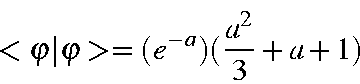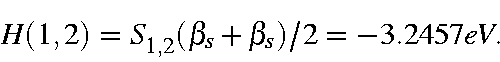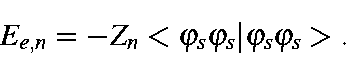# An SCF Calculation

Semiempirical calculations can be run on computers using readily available programs such as MOPAC. It is possible to use MOPAC for research without having any knowledge of its workings. This does not imply any failing on the part of the researcher; after all, it is possible to write extensive computer programs while having little knowledge of how a computer works. However, in order to more efficiently use MOPAC, a more than casual knowledge of the theory involved is desirable. In the following section the details of a very simple calculation will be described. This calculation can be carried out as a 'black box' calculation, but the following exercise may help to satisfy the intellectual curiosity of users of semiempirical methods regarding the mechanics of carrying out an SCF calculation.

The MNDO method will be used because it is the oldest of the "NDO" methods. The CNDO/2 method is very similar, and the example we will look at will emphasize the similarity. The system to be examined is a regular hexagon of hydrogen atoms in which the H-H distance is 0.98316 Ångstrom. Of course, a regular hexagon of hydrogen atoms is not a stable system; the only reason we are using it here is to demonstrate the working of an SCF calculation. The optimized geometry was obtained by defining all bond lengths to be equal, constraining all bond angles to be 120 degrees and defining the system as being planar. We will need various reference data in order to follow   the calculation. MOPAC contains a large data-set, BLOCK.F, of atomic and diatomic parameters for all the elements which have been parameterized. By reference to this source file we find that, for hydrogen:

 Gss = <φsφs|1/r|φsφs> = 12.848 eV Uss = <φs|H|φs> = -11.906276 eV ξs = 1.331967 Bohr βs = - 6.989064 eV Eatom = 52.102 kcal/mol

This exercise is designed to allow the reader to reproduce each step. All that is needed in order to follow the working is a hand calculator.

Interatomic Distance Matrix (Å)
 Atom 1 2 3 4 5 6 1 0 2 0.9832 0.0000 3 1.7029 0.9832 0.0000 4 1.9663 1.7029 0.9832 0.0000 5 1.7029 1.9663 1.7029 0.9832 0.0000 6 0.9832 1.7029 1.9663 1.7029 0.9832 0.0000

The overlap integral of two Slater orbitals between two    hydrogen atoms is particularly simple:where a = ξR/ao.

At the optimum H-H distance of 0.9831571Å, this yields an overlap integral of 0.4643. The nearest-neighbor one-electron integral is thusIn general, overlap integrals are more complicated and also involve angular components, but the principles involved are the same. You may want to check other off-diagonal terms in the one-electron matrix, or you may accept the results given here.

One-electron matrix (eV)
 Atom 1 2 3 4 5 6 1 -51.7124 2 -3.2457 -51.7124 3 -1.097 -3.2457 -51.7124 4 -0.6992 -1.0970 -3.2457 -51.7124 5 -1.097 -0.6992 -1.0970 -3.2457 -51.7124 6 -3.2457 -1.0970 -0.6992 -1.0970 -3.2457 -51.7124

On-diagonal one-electron integrals are more complicated than the off-diagonal terms. The one-electron energy of an electron in an atomic orbital is the sum of its kinetic energy and stabilization due to the positive nucleus of its own atom, Uss or Upp, plus the stabilization due to all the other nuclei in the system. Each electron on a hydrogen atom experiences a stabilization due to the five other unipositive nuclei in the system. Within semiempirical theory the electron-nuclear interaction is related to the electron-electron interaction viaGiven the two-electron two-center integral matrix the calculation of the diagonal terms of the one-electron matrix is straightforward:

Hn,n = -11.9063 - 2(9.6585) - 2(7.0635) -6.3622 = -51.7124.

For interactions between an atomic orbital and a non-hydrogen atom there will be ten terms; these arise from all permutations of the basis set, s, px, py, pz with the atomic orbital under the neglect of differential overlap approximation. The ten integrals are <ii|ss>, <ii|spx>, <ii|pxpx>, <ii|spy>, <ii|pxpy>,<ii|pypy>, <ii|spz>, <ii|pxpz>, <ii|pypz>, and <ii|pzpz>.

Two-Electron Integrals (eV)
 Atom 1 2 3 4 5 6 1 12.848 2 9.6585 12.8480 3 7.0635 9.6585 12.8480 4 6.3622 7.0732 9.6585 12.8480 5 7.0635 6.3622 7.0732 9.6585 12.8480 6 9.6585 7.0635 6.3622 7.0732 9.6585 12.8480